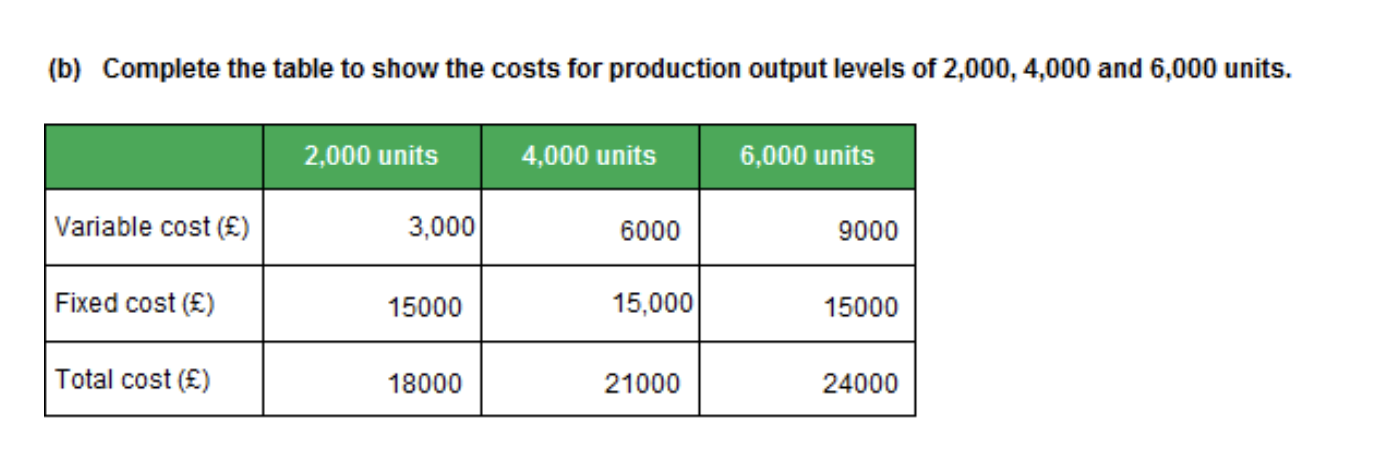# High/low Method - ELCO Mock assessment 1 - Task 6

I'm prepping for my ELCO exam this week and just have one item that I need to fully understand from a mock asessment if anyone can help?

Mock assessment 1 - Task 6 B
I understand the hi low method and that I need use a high total cost value and a low total cost value, but in this question I can only calculate one in the first column.

I've tried to use the CPU from the first column to calculate total costs for the others (CPU X units + fixed) but it doesnt match the answers below. I realise the formula is actually VCU X Units + fixed = total cost, but im not sure what else to do.

Workings:

18000 / 2000 = 9 (cost per unit)

9 (CPU) x 4000 + 15000 = 51000 (should be 21000)
9 (CPU) x 6000 + 15000 = 69000 (should be 24000)

What am I missing here?

Any help would be much appreciated

Question